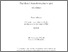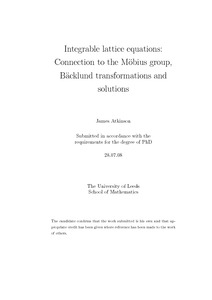# Integrable lattice equations: connection to the Möbius group, Bäcklund transformations and solutions.

Atkinson, James (2008) Integrable lattice equations: connection to the Möbius group, Bäcklund transformations and solutions. PhD thesis, University of Leeds.Preview
Text
thesis.pdf - Final eThesis - complete (pdf)
Available under License Creative Commons Attribution-Noncommercial-Share Alike 2.0 UK: England & Wales.

Download (590Kb) | Preview

## Abstract

We consider scalar integrable lattice equations which arise as the natural discrete counterparts to KdV-type PDEs. Several results are reported. We identify a new and natural connection between the ‘Schwarzian’ (Möbius invariant) integrable lattice systems and the Möbius group itself. The lattice equation in some sense describes dynamics of fixed-points as they change under composition between transformations. A classification result is given for lattice equations which are linear but also consistent on the cube. Such systems lie outside previous classification schemes. New Bäcklund transformations (BTs) for some known integrable lattice equations are given. As opposed to the natural auto-BT inherent in every such equation, these BTs are of two other kinds. Specifically, it is found that some equations admit additional auto-BTs (with Bäcklund parameter), whilst some pairs of apparently distinct equations admit a BT which connects them. Adler’s equation has come to hold the status of ‘master equation’ among the integrable lattice equations. Solutions of this equation are derived which are associated with 1-cycles and 2-cycles of the BT. They were the first explicit solutions written for Adler’s equation. We also apply the BT to the 1-cycle solution in order to construct a soliton-type solution.

Item Type: Thesis (PhD) Integrable systems, discrete, lattice equation, Backlund transformation, Soliton The University of Leeds > Faculty of Maths and Physical Sciences (Leeds) > School of Mathematics (Leeds)The University of Leeds > Faculty of Maths and Physical Sciences (Leeds) > School of Mathematics (Leeds) > Applied Mathematics (Leeds) uk.bl.ethos.502791 Dr James Atkinson 01 Jun 2015 09:08 25 Nov 2015 13:26 http://etheses.whiterose.ac.uk/id/eprint/9081

You do not need to contact us to get a copy of this thesis. Please use the 'Download' link(s) above to get a copy.
You can contact us about this thesis. If you need to make a general enquiry, please see the Contact us page.